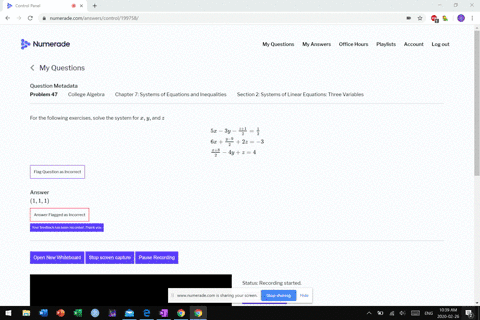Sign up for our free STEM online summer camps starting June 1st!View Summer Courses### For the following exercises, solve the system for…

05:49
MB

Need more help? Fill out this quick form to get professional live tutoring.

Get live tutoring
Problem 46

For the following exercises, solve the system for $x, y,$ and $z$
$$\begin{array}{r}{x+y+z=3} \\ {\frac{x-1}{2}+\frac{y-3}{2}+\frac{z+1}{2}=0} \\ {\frac{x-2}{3}+\frac{y+4}{3}+\frac{z-3}{3}=\frac{2}{3}}\end{array}$$

$(1.625,6.625,-3.75)$

## Discussion

You must be signed in to discuss.

## Video Transcript

right. Question 46 is the extension problem missile. The system for X y Z given fairly innocent first situation in the other two are a little bit more complex when we first see them. Um, bye. We could get written. Make these much more friendly equations fairly easily. So friends and people move by this equation Times two and moved by this equation. Times three believe the 1st 1 as it ISS. And at least that way we'll be on our way less fractions and stuff it so exposed. Wipe those three The next equation all the Tuesday cancels X minus one was why minus three. What's the UPS one people zero turns into, huh? Right that my 1st 1 again. X y z those three extra turns of the X plus y see equals. You see, I've got minus one minus that It's negative for made for close. One is negative. Three. So this equals three also so that two planes that are the same just check out what happens in this last month. So we have three planes that are all the same, so I'm all about that. But you get X minus two. Those lifeboats for was C minus three People's too case that's gonna be X plus. My Z equals negative to before it's, too. Uh, two. Minus three is negative one and one to both sides. And yet all three on the same plane, which means that there anything on the X, Y and Z is a solution. One of them uses a solution to all of them. That means there's an infinite number of solutions that nothing unique.